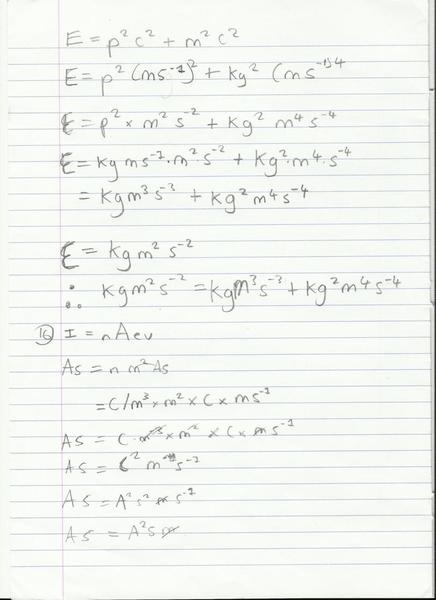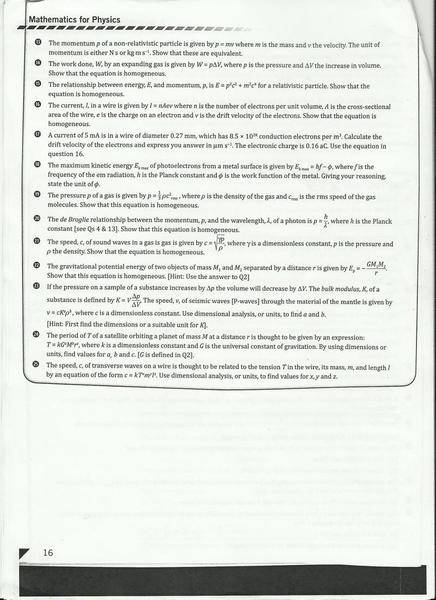MisterOrange

I have attempted two questions, see below.
1. The relationship between energy, E, and momentum, p, is E = P^2C^2 + M^2C^4 for a relativistic particle, Show that the equation is homogeneous

2. The current, I, in a wire is given by I = nAev where n is the number of electrons per unit volume, A is the cross sectional area of the wire, e is the charge on an electron and v is the drift velocity of the electrons. Show that the equation is homogeneous.

## Homework Equations

3.My attempts in photo.I am assuming 16 is definitely wrong.

and for the questions, so you can see them more articulately.MisterOrange
16 is definitely wrong.

I have since worked it.

I = A
n = m^-3
A = m^2
e = C = A*s
V = m*s^-1

which gives you A = m^2 * A * s * m * s^-1 * m^-3

Can anyone help with 17? I dont even know where to start...

Mentor
Question 15 seems to have a typo. The correct expression for the relationship between energy and momentum should have the E squared: E2 = ...

So you definitely won't be able to demonstrate that the given equation is homogenous unless this typo is fixed.

However, for the work you've show on question 15 there are some algebra issues. Each term should reduce to units the same as for energy squared. In the fourth line of your solution you "lost" the square on the momentum when you substituted in the units for momentum.

For question 16 you've made the units of n to be C/m3, but it should be just m-3 as you wrote in your summary.

willem2
16 is definitely wrong.

I have since worked it.

I = A
n = m^-3
A = m^2
e = C = A*s
V = m*s^-1

which gives you A = m^2 * A * s * m * s^-1 * m^-3

Can anyone help with 17? I dont even know where to start...

16 is ok. you're left with A = A .

17 is just using the same equation as 16. You'll have to convert all the length units to m^3 before you can use the equation, and then convert the speed you get to micrometer/s.
aC is an attocoulomb, wich i've never seen used before.